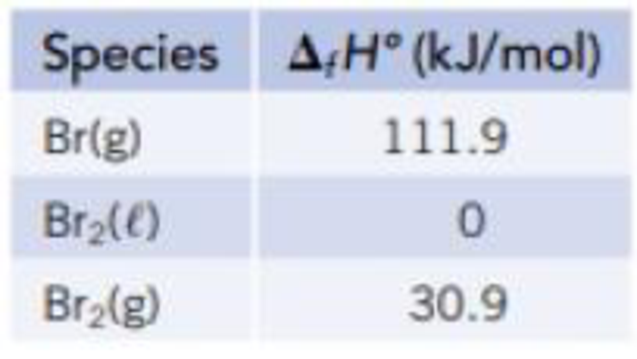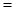Chapter 5, Problem 112SCQ

Chapter
Section
Textbook Problem

Several standard enthalpies of formation (from Appendix L) are given below. Use these data to calculate (a) the standard enthalpy of vaporization of bromine. (b) the energy required for the reaction Br2(g) → 2 Br(g). (This is the Br—Br bond dissociation enthalpy.)(a)

Interpretation Introduction

Interpretation:

The standard enthalpy of vaporization of bromine has to be determined

Concept Introduction:

The standard enthalpy of formation is the enthalpy change for the formation of 1mol of the compound directly from its component elements in their standard states.

Enthalpy change for the reaction ΔrH0ΣnΔfH0(products)-ΣnΔfH0(reactants)

Explanation

Given,

Formation constant of Br(g) is 111.9kJ/mol

Formation constant of Br2(l) is 0kJ/mol

Formation constant of Br2(g) is 30.9kJ/mol

Substitute in ΔrH0

(b)

Interpretation Introduction

Interpretation:

The energy required for the reaction of bromine has to be calculated.

Concept Introduction:

The standard enthalpy of formation is the enthalpy change for the formation of 1mol of the compound directly from its component elements in their standard states.

Enthalpy change for the reaction ΔrH0ΣnΔfH0(products)-ΣnΔfH0(reactants)

Still sussing out bartleby?

Check out a sample textbook solution.

See a sample solution

The Solution to Your Study Problems

Bartleby provides explanations to thousands of textbook problems written by our experts, many with advanced degrees!

Get Started